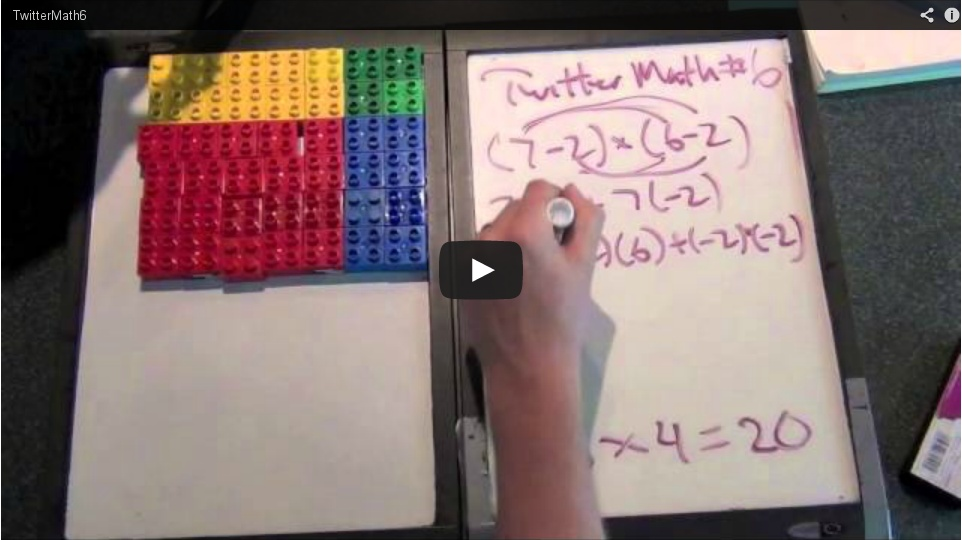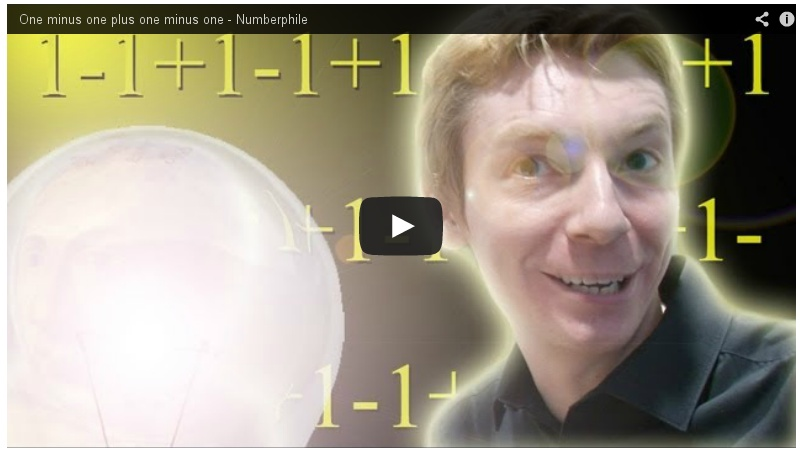# Maria's Math News, Vol. 73, July 2013

I love teaching, and I love math. This newsletter is my way of reaching out and helping you to teach it, too.Become a Better Writer Anytime, Anywhere. Write Now! www.SpiderSmart.com Real Teachers, Real results 703-943-6969 eLearning@spidersmart.com

Hello again!

For most of us, it is summer, and school is out... (though for some of you it is winter!). Yet, I have found some interesting things - hopefully for ALL of you, whether you teach elementary, middle, or high school level math. I always strive to provide a "mixed" bag of resources - though, at the same time, I fully realize I don't always succeed.

~Maria

1. A FUN measuring activity (grades 3-6)
2. Negative times negative is positive — once again! (grades 6-8)
3. Worksheets for writing & evaluating expressions (grades 6-9)
4. The series of plus 1, minus 1, plus 1, minus 1 (grades 6-12)
5. Epilogue

## 1. A FUN measuring activity (grades 3-6)

Miss MathDork has this really fun measuring activity. And it's not only fun, it ALSO teaches CONCEPTS, which is SO important in math.

Just like my hubby said... if you only focus on memorizing the formulas of math, you might get by for a little while, but eventually you come to a stopping point and it all starts falling apart.

It's kind of like having lots of "head knowledge", which won't work. You have to get "heart knowledge" - a true understanding of math and its principles and WHY it works. That's the only true way to success.

Anyway, I feel Miss MathDork is on the right track and really achieves that with her activity. She also has a poster for you to download.

r>## 2. Negative times negative is positive — once again! (grades 6-8)

In the video below, Mike shows a fun, geometric way for kids to see that a negative times negative is positive.I had not seen this exact proof before (or if I have, I had forgotten) - so it was cool to see it. Another cool thing was how it connects with the distributive property.

This illustrates two things I love about math:
1. You learn some principle (such as that negative times negative = positive). Later, you will see that same principle illustrated and proven in other contexts, over and over and over.

This is what Mike did for me here: prove a principle I already knew, once again. When this happens to your kids (they see a principle reproven), it helps them BELIEVE and remember that particular principle of mathematics.

2. I also saw how this principle I already knew CONNECTS with another principle of mathematics, this time with the distributive property. This is also what you should strive for in math teaching: to connect things students already know with new things. It's like connecting two puzzle pieces, and seeing a new picture form.

Essentially, Mike first goes through an example of multiplying (5 + 2) × (4 + 2), either by adding first (you get 7 × 6), or by multiplying the parts first, and lastly adding.

Then, he changes the example so it has negative numbers: (7 − 2) × (6 − 2). Subtracting first, then multiplying, we get 5 × 4 = 20.

But if we multiply in parts, we get 7 × 6 + 7 × (-2) + (-2) × 6 + (-2) × (-2). This HAS to equal 20, as well. The various parts come up to 42 + (-14) + (-12) and whatever (-2)  × (-2) equals.

Since 42 + (-14) + (-12) equals 16, then (-2) × (-2) must equal POSITIVE 4 so that the total ends up being 20.

Using colorful blocks gives it an additional nice 'touch'.

## 3. Worksheets for writing & evaluating expressions (grades 6-9)

I have created two more worksheet generators.
1. Free worksheets for writing expressions.
This one makes problems that give the student an expression in words, such as the quotient of 7t and 5, the difference of x and 8, divided by 2, or the quantity 8 plus 2t, cubed, and ask the student to write a mathematical expression to match that.

These worksheets suit best grades 6, 7, and 8, including pre-algebra and algebra 1 courses.

When using the actual generator, you can control the number of problems, workspace, border around the problems, and additional instructions.

Here are some quick links for ready worksheets. Refresh the worksheet page to get another of the same kind, until you are happy with the problems.
One operation; 12 problems per sheet
Two operations; 9 problems per sheet
Two or three operations; 9 problems per sheet

2. Worksheets for evaluating simple expressions (finding the value of an expression) when the value(s) for the variable(s) are given.

This is a skill practiced in grades 6-8, and specifically in pre-algebra and algebra 1 courses.

The worksheets look kind of like this:

Here are some quick links. Refresh the worksheet page to get another of the same kind, until you are happy with the problems.
Level 1: usually one operation, no negative numbers in the expressions
Level 1: usually one operation, variable may be negative/positive integer
Level 1: usually one operation, variables and the constant may be negative/positive integers

Levels 1 & 2: variables have positive integer values
Levels 1 & 2: variables and constants are negative or positive integers
Levels 1 & 2: variables and constant are positive and may have one decimal digit

Levels 2 & 3: variables and constants are positive integers
Levels 2 & 3: some variables and constant may be negative integers
Levels 2 & 3: variables and constant may be negative and may have one decimal digit

## 4. The series of plus 1, minus 1, plus 1, minus 1 (grades 6-12)

I greatly enjoyed watching the movie below, by Dr. James Grime, also known as the numberphile (hat tip goes to my hubby again!).Dr. James takes the infinite sum (series) of alternating ones and minus ones:

1 − 1 + 1 − 1 + 1 − 1 + 1 − 1 + 1 ...

Does this series have a SUM?

Curiously, if we place parenthesis into it this way:

(1 − 1) + (1 − 1) + (1 − 1) + (1 − 1) + ...

we clearly get ZERO as the sum.

BUT if we place parenthesis into it in a different way:

1 + (− 1 + 1) + (−1 + 1) + (−1 + 1) + (−1 + 1) + ...

we get ONE  as the sum!

WHAT IS GOING ON?

James also shows TWO WAYS of obtaining the sum of 1/2 for this series (see the video and wikipedia page on Grandi's series).

To get some SANITY into the situation, we need to look at the actual DEFINITION for a SUM of an infinite series.

That has to do with the partial sums. We need to look at the partial sums (that is, the sum of the first 2 terms, the sum of the first 3 terms, the sum of the first 4 terms, and so on).

IF those sums tend towards some certain number (a limit), THEN that is the SUM for the entire series!

The partial sums for Grandi's series are:

S2 = 1 − 1 = 0
S3 = 1 − 1 + 1 = 1
S4 = 1 − 1 + 1 − 1 = 0
S5 = 1 − 1 + 1 − 1 + 1 = 1
S6 = 1 − 1 + 1 − 1 + 1 − 1 = 0
S7 = 1 − 1 + 1 − 1 + 1 − 1 + 1 = 1
...

As you can see, the partial sums never get anywhere... they form a sequence of alternating 0s and 1s.

That is PROOF that this series does not "converge", or have a definite sum. Instead, it simply diverges.

But, it FOOLS you for a while, doesn't it? It's only when we put the truth of mathematics ON it, that we can see... no, it's not a nice, convergent series with a sum.

It's kind of like, you look at it one way, and you get 0. You look at it another way, and you get 1. You look at it in a few other different ways and get 1/2. It's white, it's black, it's gray... can't tell what it is.

And the series itself "jumps all over the place" with its 1s and negative 1s, never making up its mind. It's like a PRETENDER! Pretending to be a TRUE convergent series... but it is not.

For a comparison, here's a TRUE convergent series (it has a well-defined sum):

1 + 1/2 + 1/4 + 1/8 + 1/16 + 1/32 + 1/64 + ...

(It is a simple geometric series)

Its partial sums are:
S1 = 1
S2 = 1 + 1/2 = 1 1/2
S3 = 1 1/2 + 1/4 = 1 3/4
S4 = 1 3/4 + 1/8 = 1 7/8
S5 = 1 7/8 + 1/16 = 1 15/16
S6 = 1 15/16 + 1/32 = 1 31/32
S7 = 1 31/32 + 1/64 = 1 63/64
S8 = 1 63/64 + 1/128 = 1 127/128
...
These tend to 2. So, the sum of this series is 2. A true series!

## 5. Epilogue

Feel free to forward this issue to a friend/colleague! Subscribe here.

Till next time,
Maria Miller

--